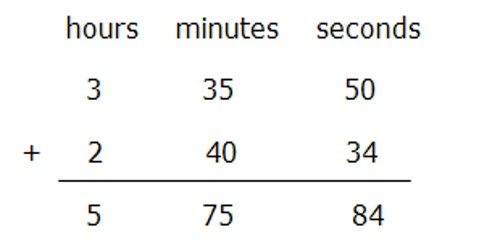In general, summing or subtracting time will get the time format such as hh:mm:ss, but in some cases, you just want to sum or subtract time to get hours or minutes or seconds only, how can you quickly handle it.

If the number of seconds is 60 or more do the following:

• Subtract 60 from the number of seconds.
• Increase the number of minutes by one.
• Repeat this process if the number of seconds is still 60 or more.Example: Add 4 minutes 21 seconds plus 2 minutes 47 seconds.

Add the minutes together. (4 minutes + 2 minutes = 6 minutes)

Add the seconds together. (21 seconds + 47 seconds = 68 seconds)

If the number of seconds is 60 or more do the following:

• Subtract 60 from the number of seconds. (68 seconds – 60 seconds = 8 seconds)
• Increase the number of minutes by one. (6 minutes + 1 minute = 7 minutes)
• Repeat this process if the number of seconds is still 60 or more.